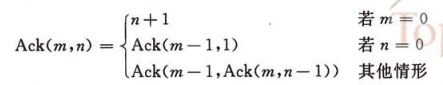# 数据结构重读 – 递归与汉诺塔

Fib(n) = Fib(n-1) + Fib(n-2) (n>=2) Fib(0) = 0 Fib(1) = 1

Fibnacci数列：1, 1, 2, 3, 5, 8, 13, 21, ....```#include <stdio.h>

int move(int n, char src, char dst)
{
printf("move %d from %c to %c \n", n, src, dst);
}

// Move disk #n from x to z with help of y
void hanoi(int n, int x, int y, int z)
{
if(n==1)
{
move(1, x, z);
}else
{
hanoi(n-1, x, z, y);
move(n, x, z);
hanoi(n-1, y, x, z);
}
}

int main()
{
hanoi(3, 'x', 'y', 'z');
return 0;
}```

move 1 from x to y
move 2 from x to z
move 1 from y to z
move 3 from x to y
move 1 from z to x
move 2 from z to y
move 1 from x to y
move 4 from x to z
move 1 from y to z
move 2 from y to x
move 1 from z to x
move 3 from y to z
move 1 from x to y
move 2 from x to z
move 1 from y to z

1、先调用先返回的原则

2、调用函数和被调用函数之间的链接和信息交换要通过栈来进行。

3、没进入一层递归，就产生一个新的工作记录压入栈顶。每退出一层递归，就从栈顶弹出一个工作记录。当前正在执行的叫做活动记录。